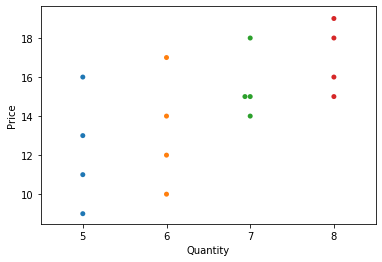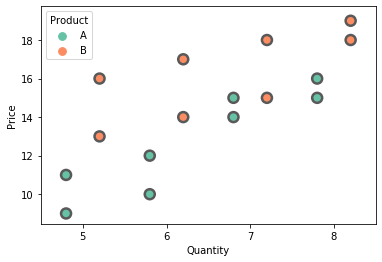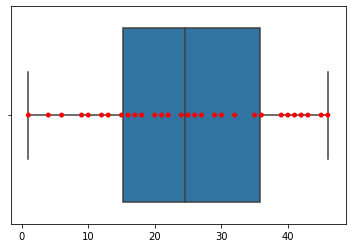# Seaborn Swarmplot

``````import pandas as pd
import matplotlib.pyplot as plt
import seaborn as sns
df = pd.DataFrame({"Quantity": [5,6,7,8,5,6,7,8,5,6,7,8,5,6,7,8],
"Price": [9,10,15,16,13,14,15,18,11,12,14,15,16,17,18,19],
"Day" : [1,1,1,1,1,1,1,1,2,2,2,2,2,2,2,2],
"Product": ['A','A','A','A','B','B','B','B',
'A','A','A','A','B','B','B','B']})
sns.swarmplot(data = df, y = "Price", x = "Quantity")
````````````import pandas as pd
import matplotlib.pyplot as plt
import seaborn as sns
df = pd.DataFrame({"Quantity": [5,6,7,8,5,6,7,8,5,6,7,8,5,6,7,8],
"Price": [9,10,15,16,13,14,15,18,11,12,14,15,16,17,18,19],
"Day" : [1,1,1,1,1,1,1,1,2,2,2,2,2,2,2,2],
"Product": ['A','A','A','A','B','B','B','B',
'A','A','A','A','B','B','B','B']})
sns.swarmplot(data = df, y = "Price", x = "Quantity", hue = 'Product', dodge = True,
linewidth = 2.5 , palette = 'Set2', size  = 10)
```````swarmplot()` 也可以用作对盒子或小提琴图的很好的赞美。它可以很好地了解数据的分布。

``````import random
import numpy as np
import seaborn as sns
n = random.sample(range(0,50),30)
arr = np.array(n)
sns.boxplot(n)
sns.swarmplot(n, color = 'red')
```````catplot()` 函数也可以创建这样的群图。为此，我们需要将 `catplot()` 函数中 `kind` 参数的值指定为 `swarm`